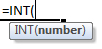### INT Function Examples – Excel, VBA, & Google Sheets

This tutorial demonstrates how to use the Excel INT Function in Excel to round a number down to the nearest integer (truncate the number).

## INT Function Overview

The INT Function Rounds a number down to the nearest integer.

To use the INT Excel Worksheet Function, select a cell and type:(Notice how the formula inputs appear)

### INT Function Syntax and Inputs:

`=INT(number)`

number – A number.

AutoMacro - VBA Code Generator

The INT Function works exactly the same in Google Sheets as in Excel:

## INT Examples in VBA

You can also use the INT function in VBA. Type:
`application.worksheetfunction.int(number)`
For the function arguments (number, etc.), you can either enter them directly into the function, or define variables to use instead.